Research papers and code for "Jian Li":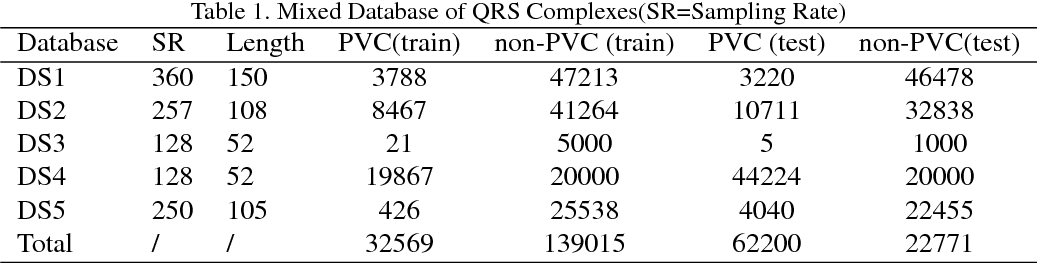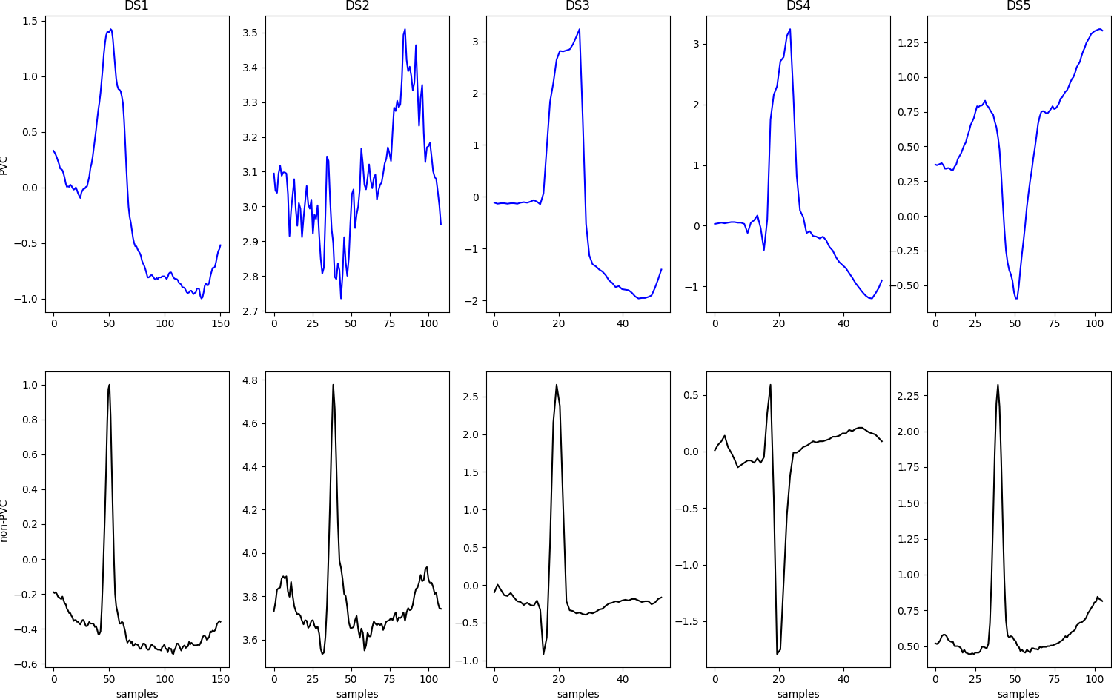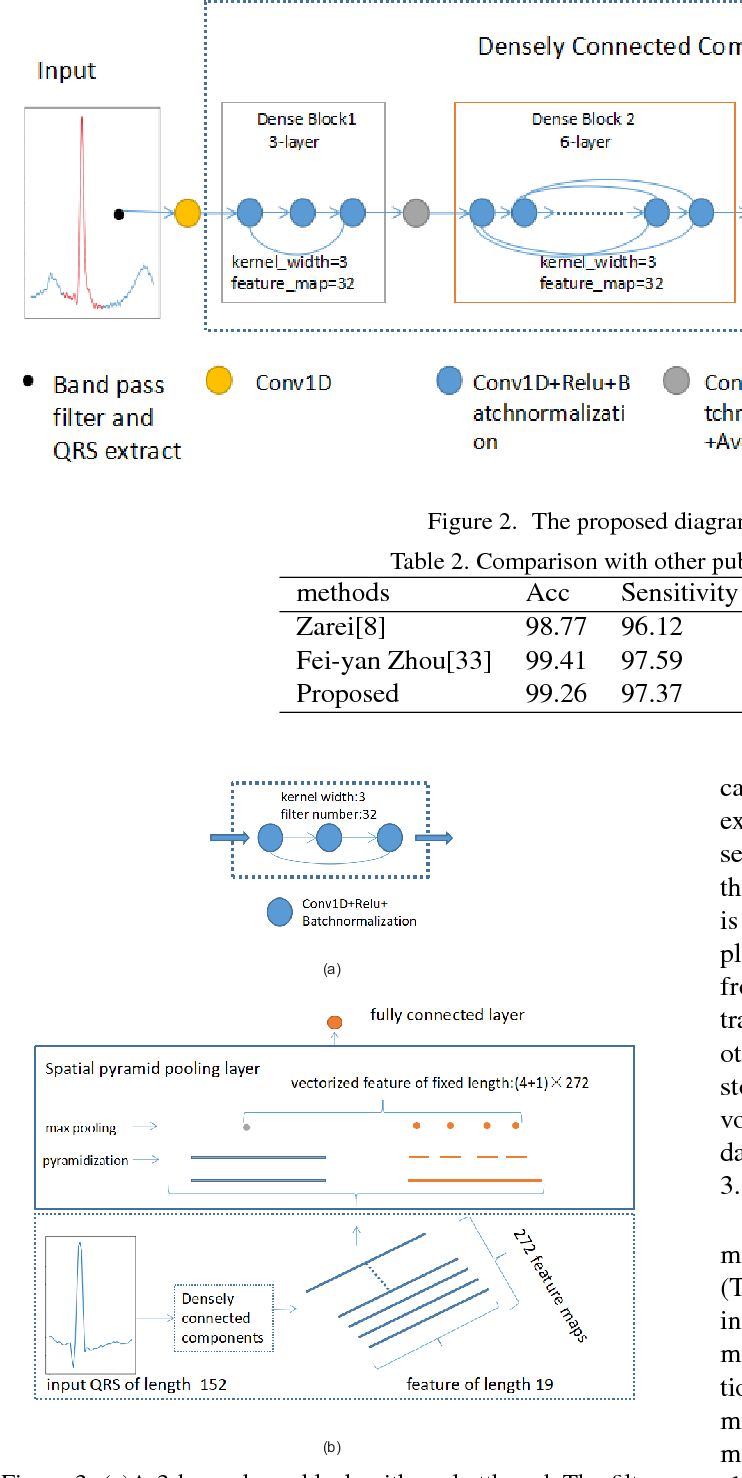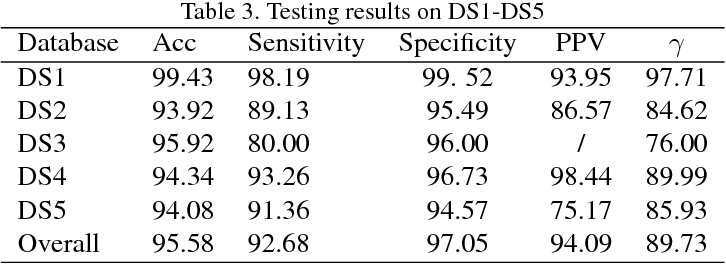Premature ventricular contraction(PVC) is a type of premature ectopic beat originating from the ventricles. Automatic method for accurate and robust detection of PVC is highly clinically desired.Currently, most of these methods are developed and tested using the same database divided into training and testing set and their generalization performance across databases has not been fully validated. In this paper, a method based on densely connected convolutional neural network and spatial pyramid pooling is proposed for PVC detection which can take arbitrarily-sized QRS complexes as input both in training and testing. With a much less complicated and more straightforward architecture,the proposed network achieves comparable results to current state-of-the-art deep learning based method with regard to accuracy,sensitivity and specificity by training and testing using the MIT-BIH arrhythmia database as benchmark.Besides the benchmark database,QRS complexes are extracted from four more open databases namely the St-Petersburg Institute of Cardiological Technics 12-lead Arrhythmia Database,The MIT-BIH Normal Sinus Rhythm Database,The MIT-BIH Long Term Database and European ST-T Database. The extracted QRS complexes are different in length and sampling rate among the five databases.Cross-database training and testing is also experimented.The performance of the network shows an improvement on the benchmark database according to the result demonstrating the advantage of using multiple databases for training over using only a single database.The network also achieves satisfactory scores on the other four databases showing good generalization capability.

* 7 figures, 4 Tables
Click to Read Paper and Get Code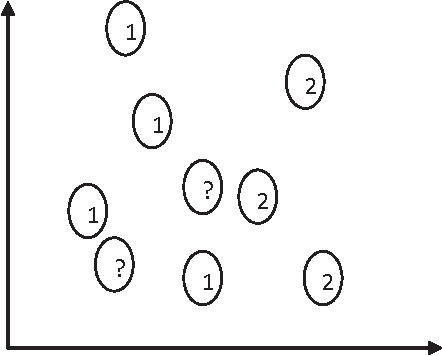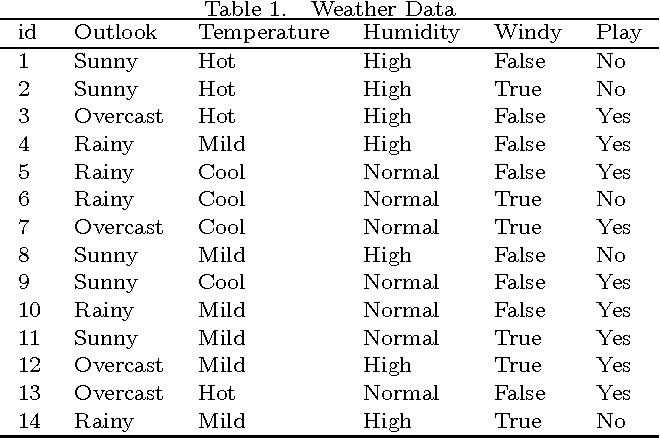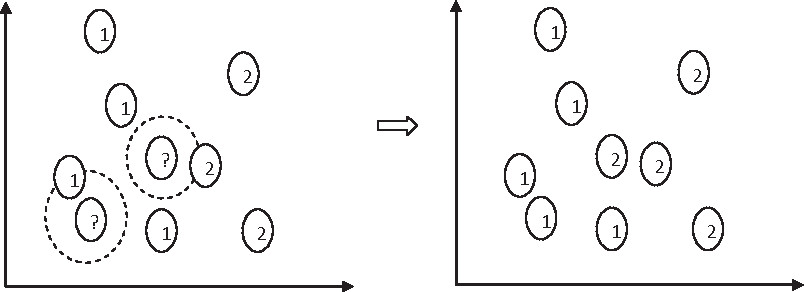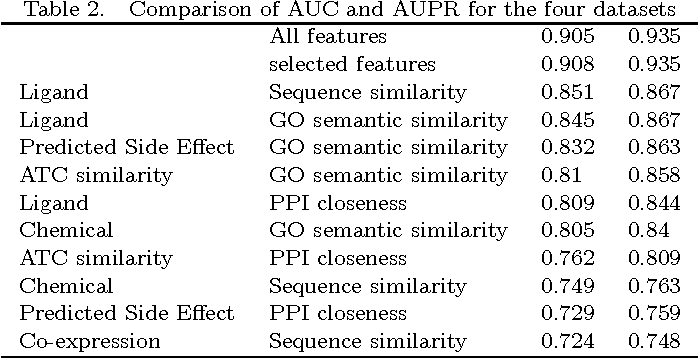Classification is one of the most popular and widely used supervised learning tasks, which categorizes objects into predefined classes based on known knowledge. Classification has been an important research topic in machine learning and data mining. Different classification methods have been proposed and applied to deal with various real-world problems. Unlike unsupervised learning such as clustering, a classifier is typically trained with labeled data before being used to make prediction, and usually achieves higher accuracy than unsupervised one. In this paper, we first define classification and then review several representative methods. After that, we study in details the application of classification to a critical problem in drug discovery, i.e., drug-target prediction, due to the challenges in predicting possible interactions between drugs and targets.

Click to Read Paper and Get Code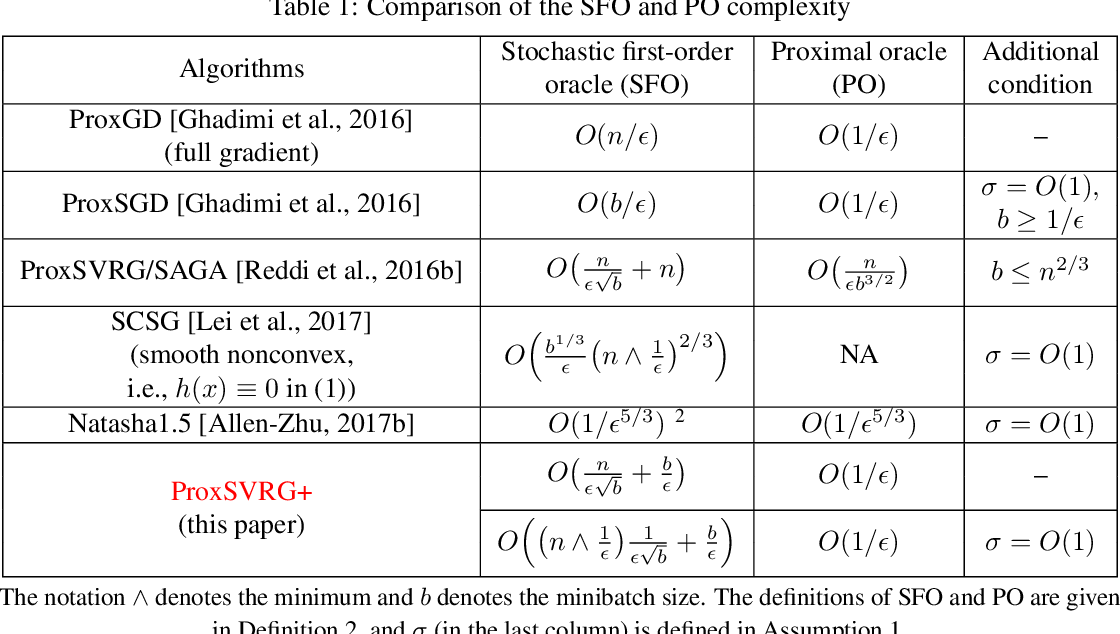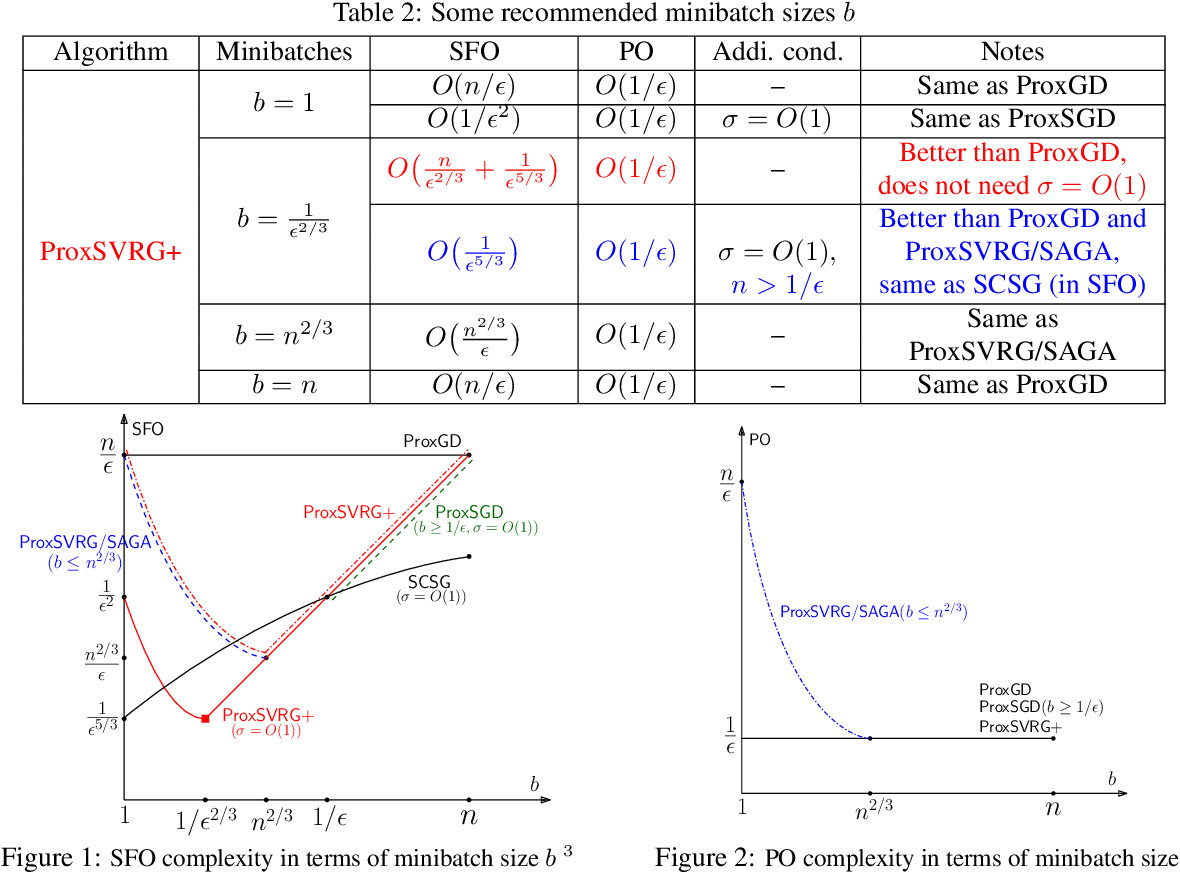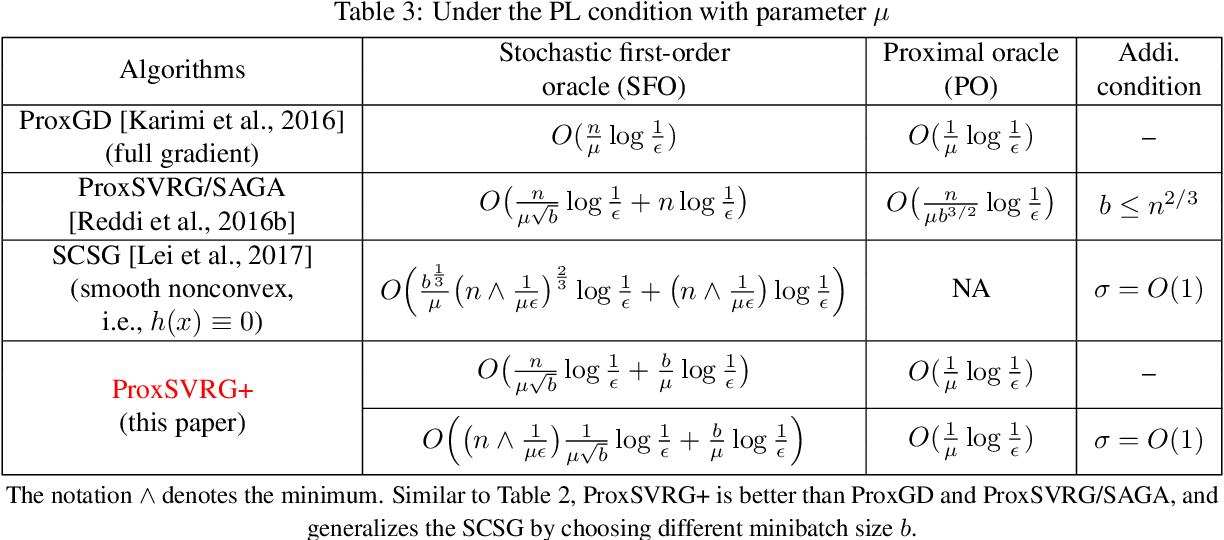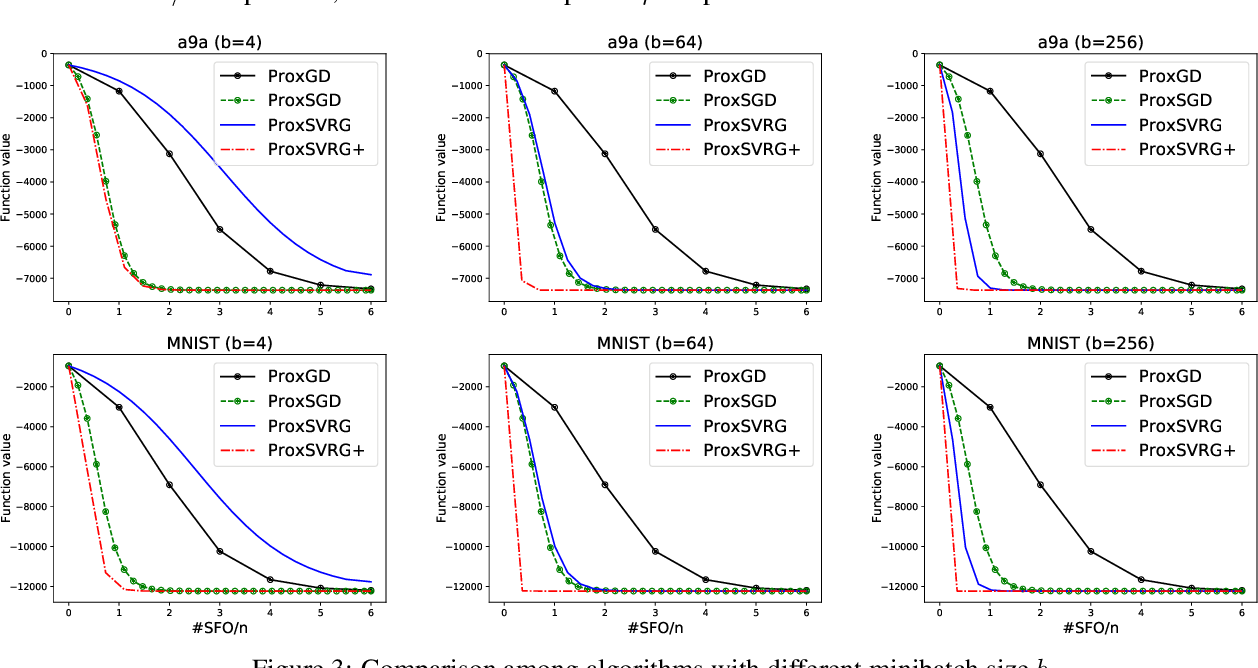We analyze stochastic gradient algorithms for optimizing nonconvex, nonsmooth finite-sum problems. In particular, the objective function is given by the summation of a differentiable (possibly nonconvex) component, together with a possibly non-differentiable but convex component. We propose a proximal stochastic gradient algorithm based on variance reduction, called ProxSVRG+. Our main contribution lies in the analysis of ProxSVRG+. It recovers several existing convergence results and improves/generalizes them (in terms of the number of stochastic gradient oracle calls and proximal oracle calls). In particular, ProxSVRG+ generalizes the best results given by the SCSG algorithm, recently proposed by [Lei et al., NIPS'17] for the smooth nonconvex case. ProxSVRG+ is also more straightforward than SCSG and yields simpler analysis. Moreover, ProxSVRG+ outperforms the deterministic proximal gradient descent (ProxGD) for a wide range of minibatch sizes, which partially solves an open problem proposed in [Reddi et al., NIPS'16]. Also, ProxSVRG+ uses much less proximal oracle calls than ProxSVRG [Reddi et al., NIPS'16]. Moreover, for nonconvex functions satisfied Polyak-\L{}ojasiewicz condition, we prove that ProxSVRG+ achieves a global linear convergence rate without restart unlike ProxSVRG. Thus, it can \emph{automatically} switch to the faster linear convergence in some regions as long as the objective function satisfies the PL condition locally in these regions. ProxSVRG+ also improves ProxGD and ProxSVRG/SAGA, and generalizes the results of SCSG in this case. Finally, we conduct several experiments and the experimental results are consistent with the theoretical results.

* 32nd Conference on Neural Information Processing Systems (NIPS 2018)
Click to Read Paper and Get Code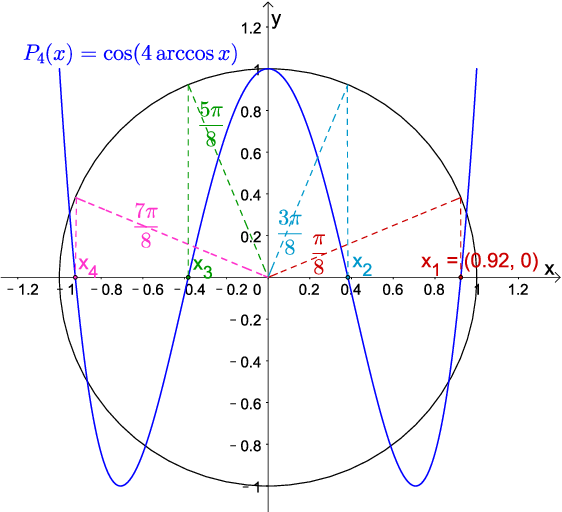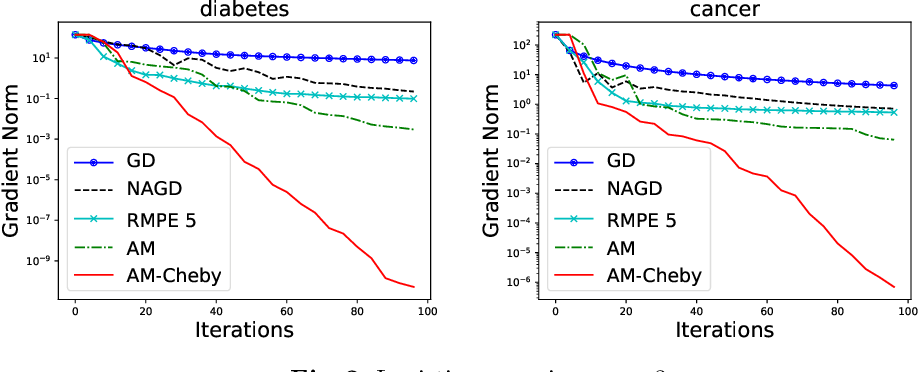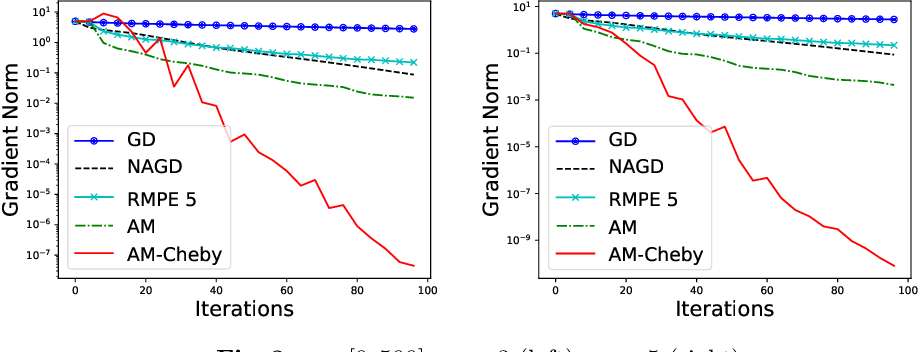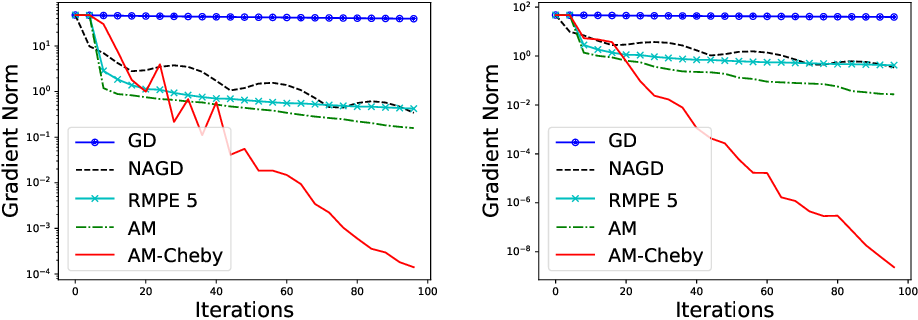Anderson mixing (or Anderson acceleration) is an efficient acceleration method for fixed point iterations (i.e., $x_{t+1}=G(x_t)$), e.g., gradient descent can be viewed as iteratively applying the operation $G(x) = x-\alpha\nabla f(x)$. It is known that Anderson mixing is quite efficient in practice and can be viewed as an extension of Krylov subspace methods for nonlinear problems. First, we show that Anderson mixing with Chebyshev polynomial parameters can achieve the optimal convergence rate $O(\sqrt{\kappa}\ln\frac{1}{\epsilon})$, which improves the previous result $O(\kappa\ln\frac{1}{\epsilon})$ provided by [Toth and Kelley, 2015] for quadratic functions. Then, we provide a convergence analysis for minimizing general nonlinear problems. Besides, if the hyperparameters (e.g., the Lipschitz smooth parameter $L$) are not available, we propose a Guessing Algorithm for guessing them dynamically and also prove a similar convergence rate. Finally, the experimental results demonstrate that the proposed Anderson-Chebyshev mixing method converges significantly faster than other algorithms, e.g., vanilla gradient descent (GD), Nesterov's Accelerated GD. Also, these algorithms combined with the proposed guessing algorithm (guessing the hyperparameters dynamically) achieve much better performance.

* 20 pages
Click to Read Paper and Get Code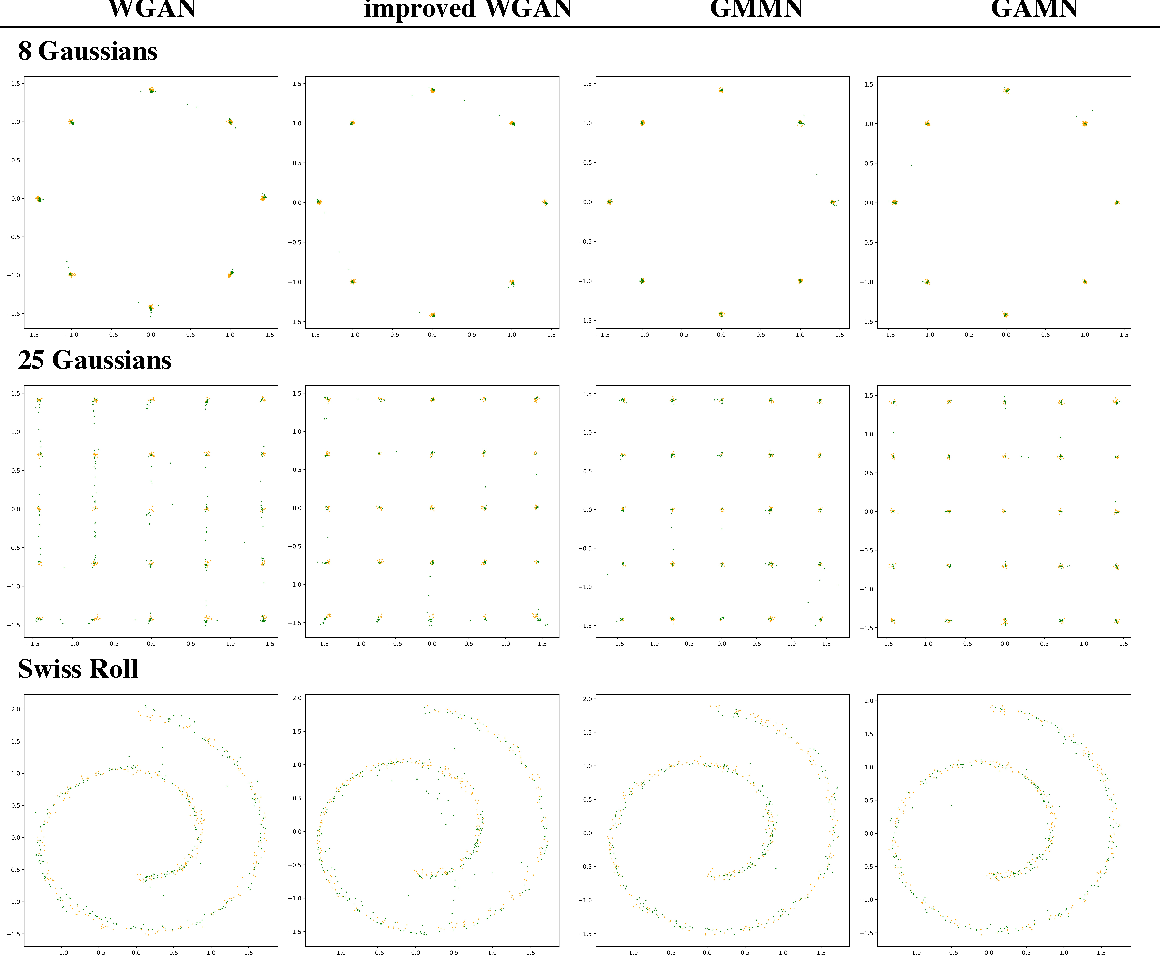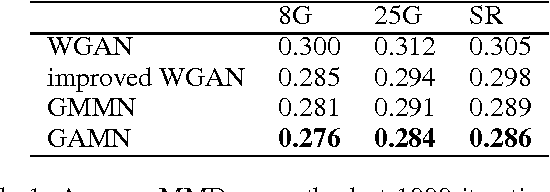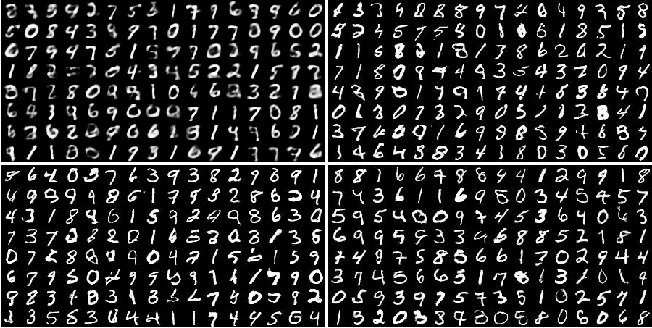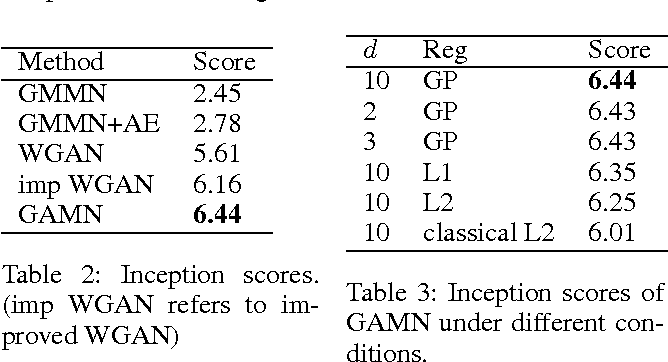Generative Adversarial Networks (GANs) have shown impressive performance in generating photo-realistic images. They fit generative models by minimizing certain distance measure between the real image distribution and the generated data distribution. Several distance measures have been used, such as Jensen-Shannon divergence, $f$-divergence, and Wasserstein distance, and choosing an appropriate distance measure is very important for training the generative network. In this paper, we choose to use the maximum mean discrepancy (MMD) as the distance metric, which has several nice theoretical guarantees. In fact, generative moment matching network (GMMN) (Li, Swersky, and Zemel 2015) is such a generative model which contains only one generator network $G$ trained by directly minimizing MMD between the real and generated distributions. However, it fails to generate meaningful samples on challenging benchmark datasets, such as CIFAR-10 and LSUN. To improve on GMMN, we propose to add an extra network $F$, called mapper. $F$ maps both real data distribution and generated data distribution from the original data space to a feature representation space $\mathcal{R}$, and it is trained to maximize MMD between the two mapped distributions in $\mathcal{R}$, while the generator $G$ tries to minimize the MMD. We call the new model generative adversarial mapping networks (GAMNs). We demonstrate that the adversarial mapper $F$ can help $G$ to better capture the underlying data distribution. We also show that GAMN significantly outperforms GMMN, and is also superior to or comparable with other state-of-the-art GAN based methods on MNIST, CIFAR-10 and LSUN-Bedrooms datasets.

* 9 pages, 7 figures
Click to Read Paper and Get Code
In the classical best arm identification (Best-$1$-Arm) problem, we are given $n$ stochastic bandit arms, each associated with a reward distribution with an unknown mean. We would like to identify the arm with the largest mean with probability at least $1-\delta$, using as few samples as possible. Understanding the sample complexity of Best-$1$-Arm has attracted significant attention since the last decade. However, the exact sample complexity of the problem is still unknown. Recently, Chen and Li made the gap-entropy conjecture concerning the instance sample complexity of Best-$1$-Arm. Given an instance $I$, let $\mu_{[i]}$ be the $i$th largest mean and $\Delta_{[i]}=\mu_{}-\mu_{[i]}$ be the corresponding gap. $H(I)=\sum_{i=2}^n\Delta_{[i]}^{-2}$ is the complexity of the instance. The gap-entropy conjecture states that $\Omega\left(H(I)\cdot\left(\ln\delta^{-1}+\mathsf{Ent}(I)\right)\right)$ is an instance lower bound, where $\mathsf{Ent}(I)$ is an entropy-like term determined by the gaps, and there is a $\delta$-correct algorithm for Best-$1$-Arm with sample complexity $O\left(H(I)\cdot\left(\ln\delta^{-1}+\mathsf{Ent}(I)\right)+\Delta_{}^{-2}\ln\ln\Delta_{}^{-1}\right)$. If the conjecture is true, we would have a complete understanding of the instance-wise sample complexity of Best-$1$-Arm. We make significant progress towards the resolution of the gap-entropy conjecture. For the upper bound, we provide a highly nontrivial algorithm which requires $O\left(H(I)\cdot\left(\ln\delta^{-1} +\mathsf{Ent}(I)\right)+\Delta_{}^{-2}\ln\ln\Delta_{}^{-1}\mathrm{polylog}(n,\delta^{-1})\right)$ samples in expectation. For the lower bound, we show that for any Gaussian Best-$1$-Arm instance with gaps of the form $2^{-k}$, any $\delta$-correct monotone algorithm requires $\Omega\left(H(I)\cdot\left(\ln\delta^{-1} + \mathsf{Ent}(I)\right)\right)$ samples in expectation.

* Accepted to COLT 2017
Click to Read Paper and Get Code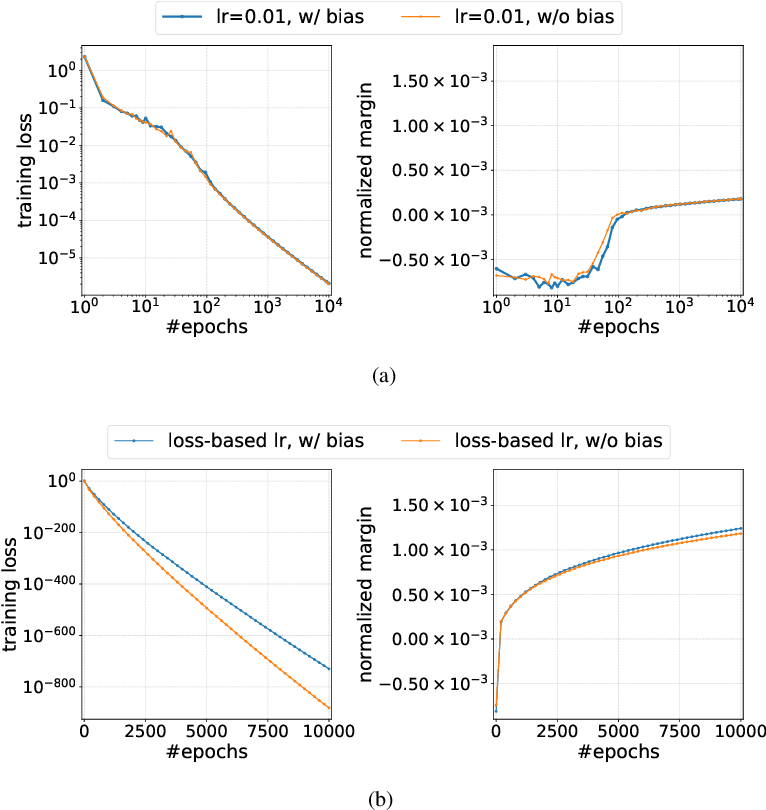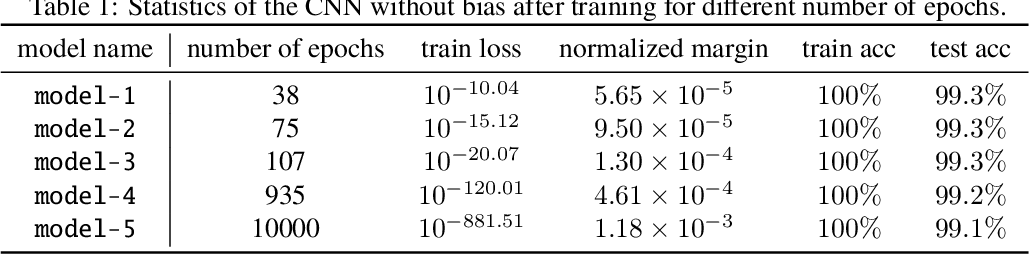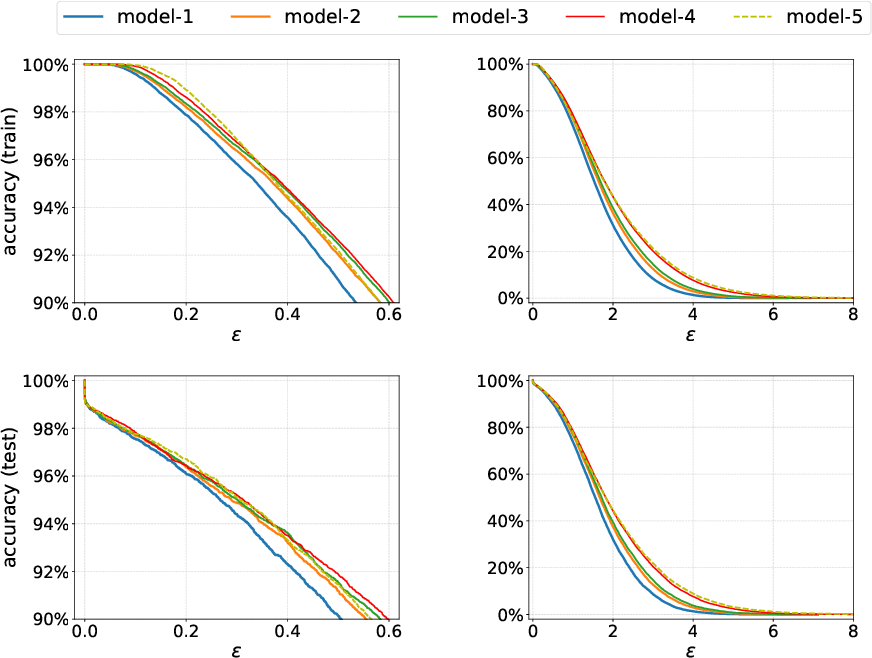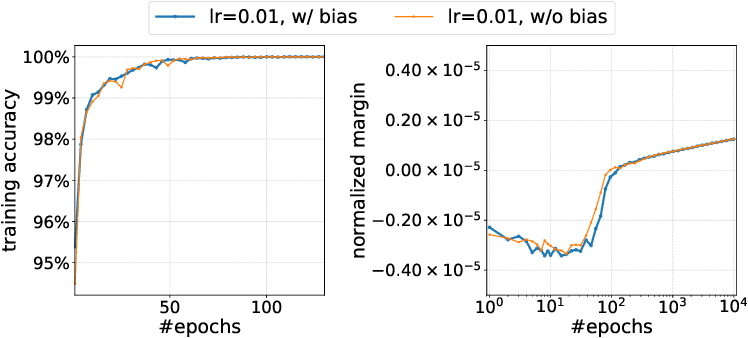Recent works on implicit regularization have shown that gradient descent converges to the max-margin direction for logistic regression with one-layer or multi-layer linear networks. In this paper, we generalize this result to homogeneous neural networks, including fully-connected and convolutional neural networks with ReLU or LeakyReLU activations. In particular, we study the gradient flow (gradient descent with infinitesimal step size) optimizing the logistic loss or cross-entropy loss of any homogeneous model (possibly non-smooth), and show that if the training loss decreases below a certain threshold, then we can define a smoothed version of the normalized margin which increases over time. We also formulate a natural constrained optimization problem related to margin maximization, and prove that both the normalized margin and its smoothed version converge to the objective value at a KKT point of the optimization problem. Furthermore, we extend the above results to a large family of loss functions. We conduct several experiments to justify our theoretical finding on MNIST and CIFAR-10 datasets. For gradient descent with constant learning rate, we observe that the normalized margin indeed keeps increasing after the dataset is fitted, but the speed is very slow. However, if we schedule the learning rate more carefully, we can observe a more rapid growth of the normalized margin. Finally, as margin is closely related to robustness, we discuss potential benefits of training longer for improving the robustness of the model.

* 35 pages, 7 figures
Click to Read Paper and Get Code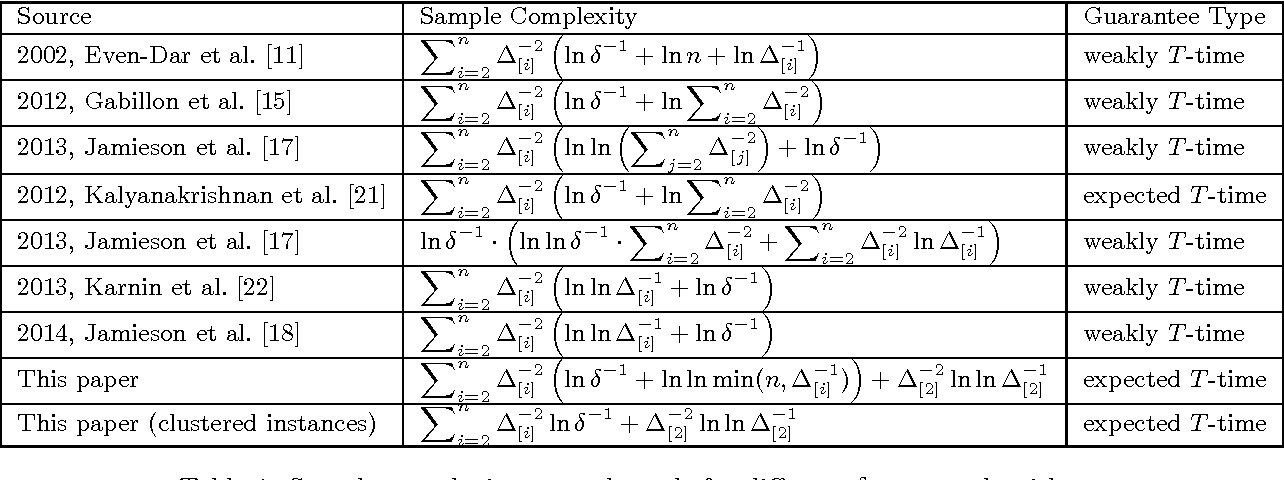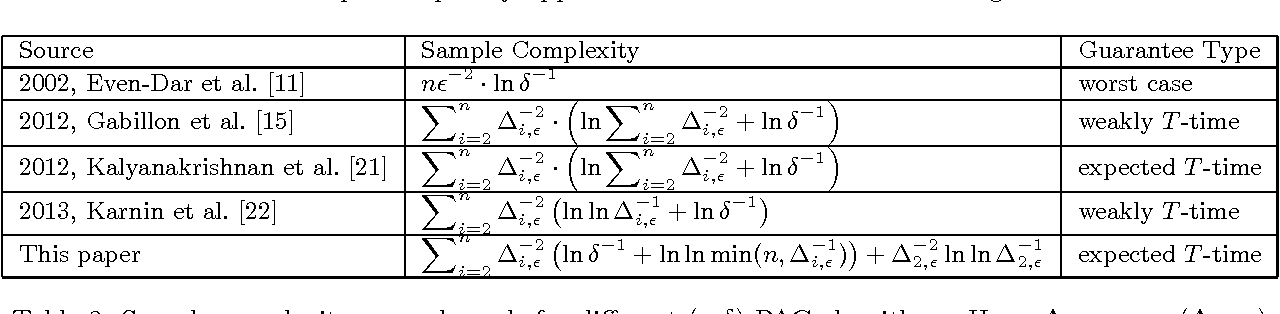We study the best arm identification (BEST-1-ARM) problem, which is defined as follows. We are given $n$ stochastic bandit arms. The $i$th arm has a reward distribution $D_i$ with an unknown mean $\mu_{i}$. Upon each play of the $i$th arm, we can get a reward, sampled i.i.d. from $D_i$. We would like to identify the arm with the largest mean with probability at least $1-\delta$, using as few samples as possible. We provide a nontrivial algorithm for BEST-1-ARM, which improves upon several prior upper bounds on the same problem. We also study an important special case where there are only two arms, which we call the sign problem. We provide a new lower bound of sign, simplifying and significantly extending a classical result by Farrell in 1964, with a completely new proof. Using the new lower bound for sign, we obtain the first lower bound for BEST-1-ARM that goes beyond the classic Mannor-Tsitsiklis lower bound, by an interesting reduction from Sign to BEST-1-ARM. We propose an interesting conjecture concerning the optimal sample complexity of BEST-1-ARM from the perspective of instance-wise optimality.

Click to Read Paper and Get Code
The best arm identification problem (BEST-1-ARM) is the most basic pure exploration problem in stochastic multi-armed bandits. The problem has a long history and attracted significant attention for the last decade. However, we do not yet have a complete understanding of the optimal sample complexity of the problem: The state-of-the-art algorithms achieve a sample complexity of $O(\sum_{i=2}^{n} \Delta_{i}^{-2}(\ln\delta^{-1} + \ln\ln\Delta_i^{-1}))$ ($\Delta_{i}$ is the difference between the largest mean and the $i^{th}$ mean), while the best known lower bound is $\Omega(\sum_{i=2}^{n} \Delta_{i}^{-2}\ln\delta^{-1})$ for general instances and $\Omega(\Delta^{-2} \ln\ln \Delta^{-1})$ for the two-arm instances. We propose to study the instance-wise optimality for the BEST-1-ARM problem. Previous work has proved that it is impossible to have an instance optimal algorithm for the 2-arm problem. However, we conjecture that modulo the additive term $\Omega(\Delta_2^{-2} \ln\ln \Delta_2^{-1})$ (which is an upper bound and worst case lower bound for the 2-arm problem), there is an instance optimal algorithm for BEST-1-ARM. Moreover, we introduce a new quantity, called the gap entropy for a best-arm problem instance, and conjecture that it is the instance-wise lower bound. Hence, resolving this conjecture would provide a final answer to the old and basic problem.

* To appear in COLT 2016 Open Problems
Click to Read Paper and Get Code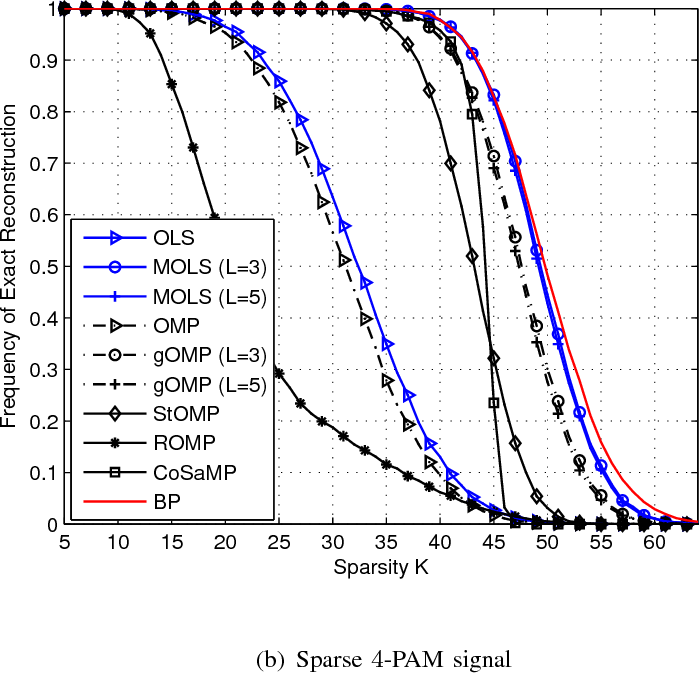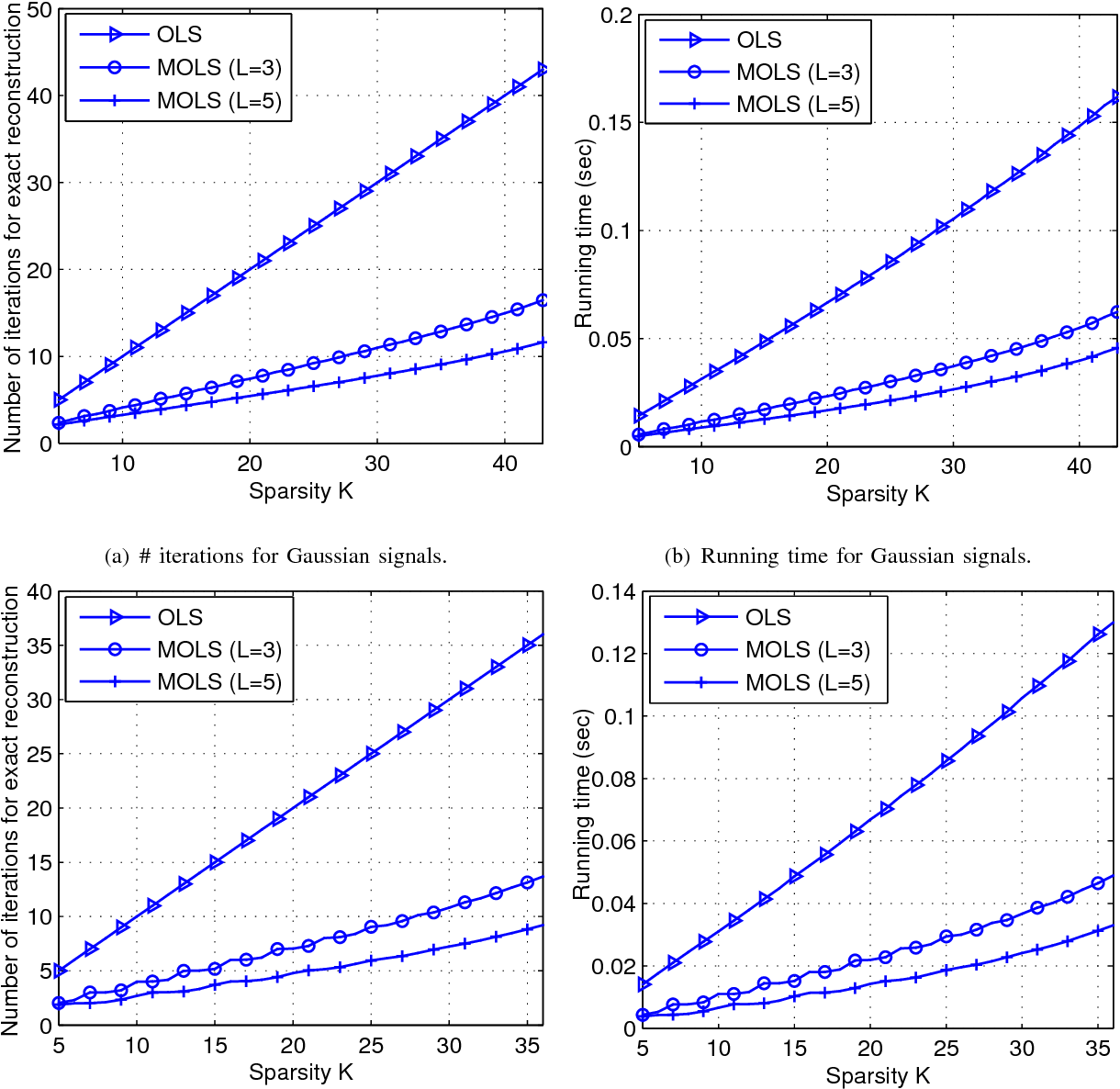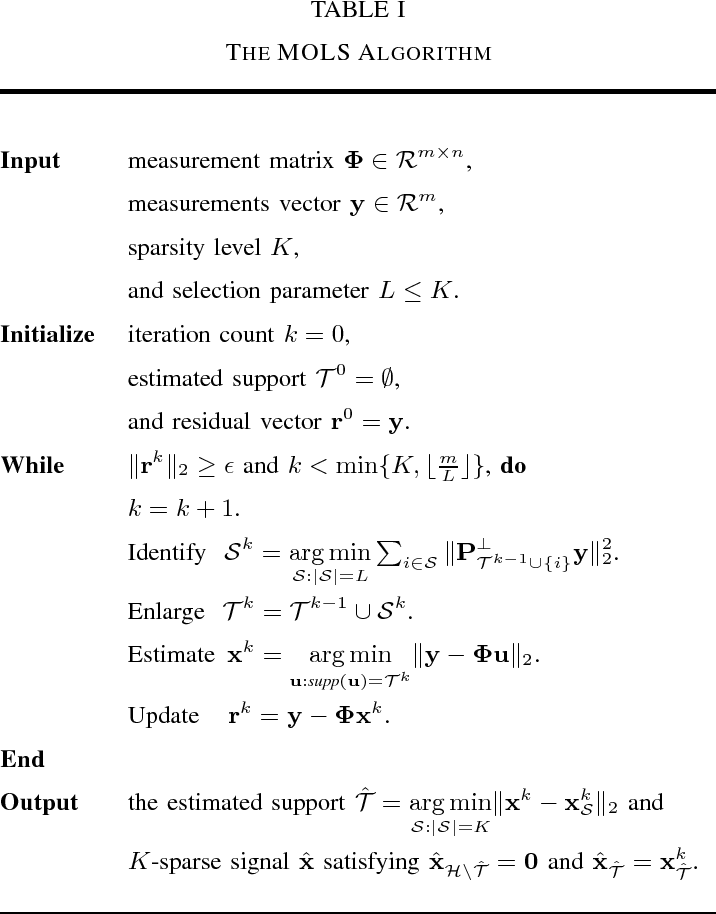We study the problem of recovering sparse signals from compressed linear measurements. This problem, often referred to as sparse recovery or sparse reconstruction, has generated a great deal of interest in recent years. To recover the sparse signals, we propose a new method called multiple orthogonal least squares (MOLS), which extends the well-known orthogonal least squares (OLS) algorithm by allowing multiple $L$ indices to be chosen per iteration. Owing to inclusion of multiple support indices in each selection, the MOLS algorithm converges in much fewer iterations and improves the computational efficiency over the conventional OLS algorithm. Theoretical analysis shows that MOLS ($L > 1$) performs exact recovery of all $K$-sparse signals within $K$ iterations if the measurement matrix satisfies the restricted isometry property (RIP) with isometry constant $\delta_{LK} < \frac{\sqrt{L}}{\sqrt{K} + 2 \sqrt{L}}.$ The recovery performance of MOLS in the noisy scenario is also studied. It is shown that stable recovery of sparse signals can be achieved with the MOLS algorithm when the signal-to-noise ratio (SNR) scales linearly with the sparsity level of input signals.

Click to Read Paper and Get Code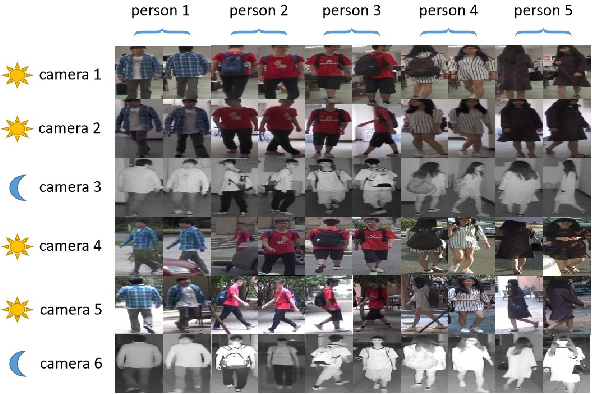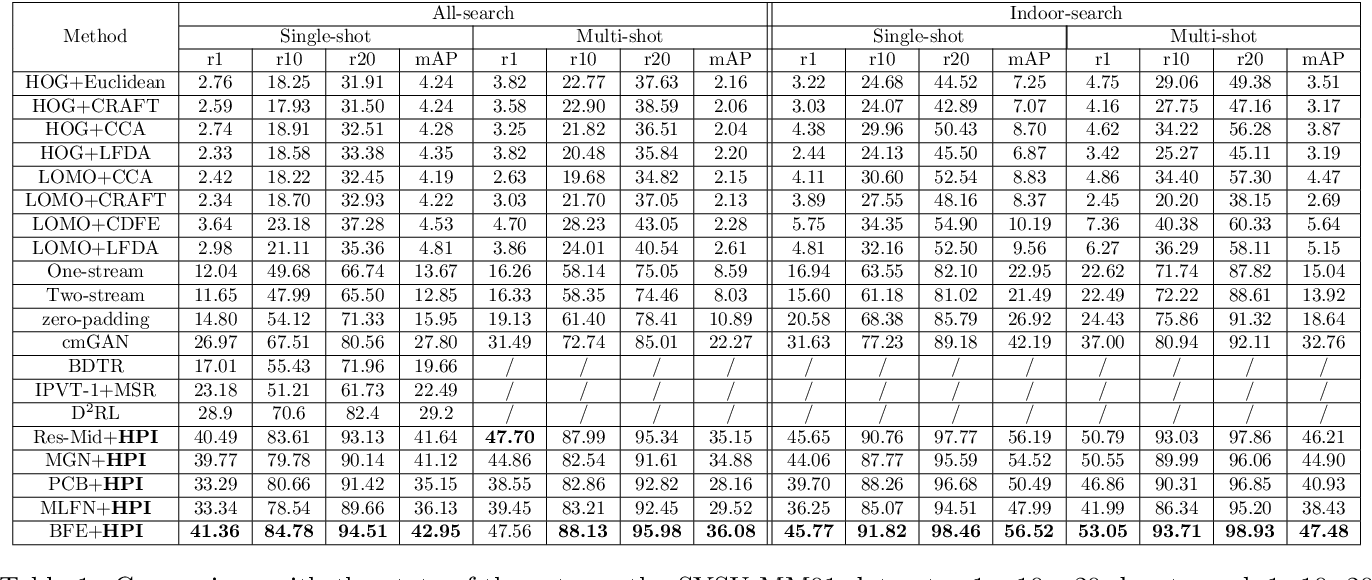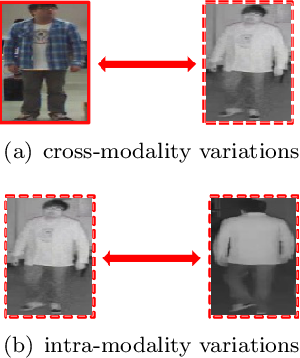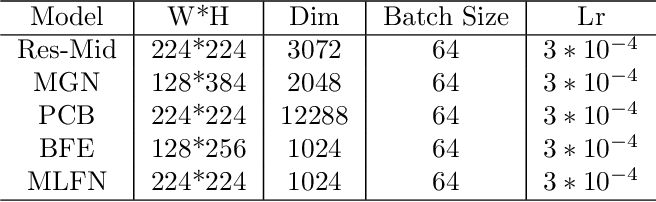Most video surveillance systems use both RGB and infrared cameras, making it a vital technique to re-identify a person cross the RGB and infrared modalities. This task can be challenging due to both the cross-modality variations caused by heterogeneous images in RGB and infrared, and the intra-modality variations caused by the heterogeneous human poses, camera views, light brightness, etc. To meet these challenges a novel feature learning framework, HPILN, is proposed. In the framework existing single-modality re-identification models are modified to fit for the cross-modality scenario, following which specifically designed hard pentaplet loss and identity loss are used to improve the performance of the modified cross-modality re-identification models. Based on the benchmark of the SYSU-MM01 dataset, extensive experiments have been conducted, which show that the proposed method outperforms all existing methods in terms of Cumulative Match Characteristic curve (CMC) and Mean Average Precision (MAP).

Click to Read Paper and Get Code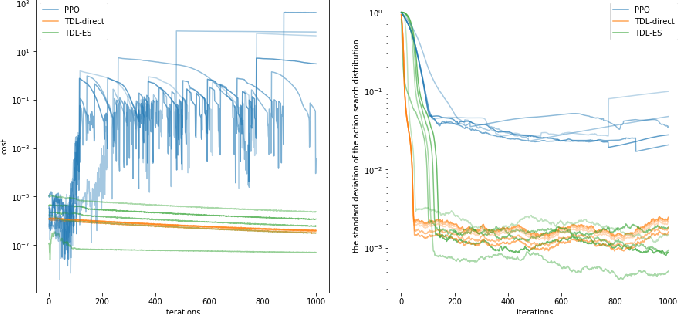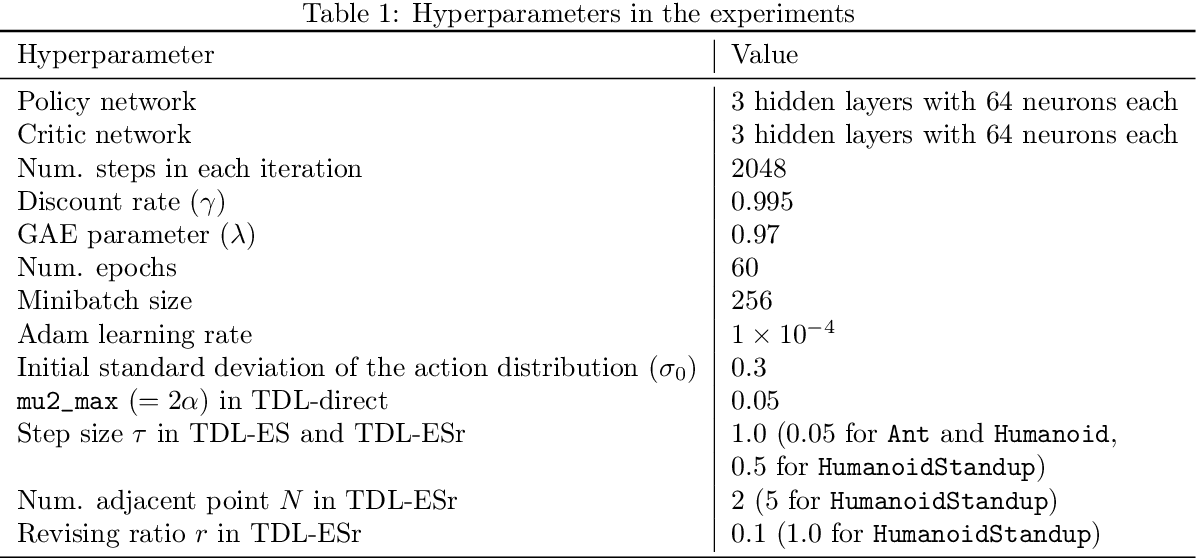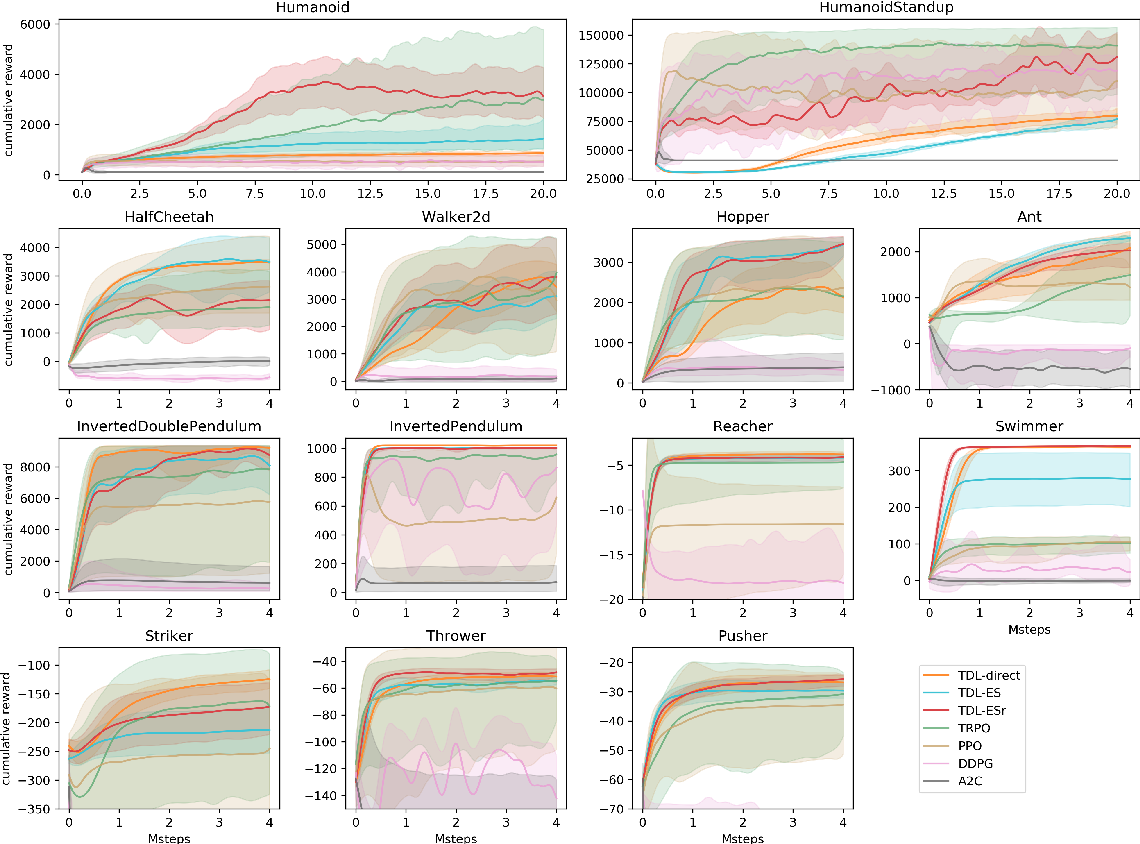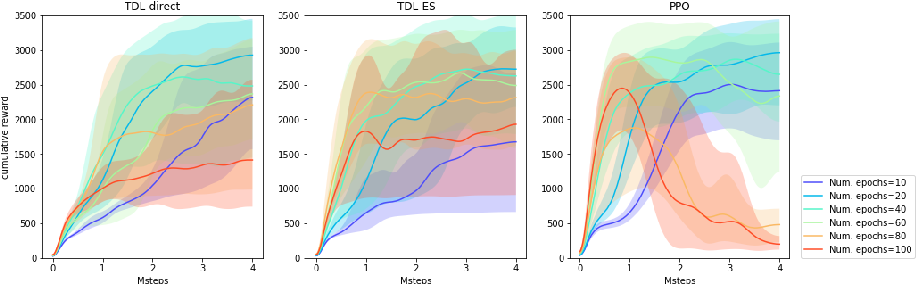We observe that several existing policy gradient methods (such as vanilla policy gradient, PPO, A2C) may suffer from overly large gradients when the current policy is close to deterministic (even in some very simple environments), leading to an unstable training process. To address this issue, we propose a new method, called \emph{target distribution learning} (TDL), for policy improvement in reinforcement learning. TDL alternates between proposing a target distribution and training the policy network to approach the target distribution. TDL is more effective in constraining the KL divergence between updated policies, and hence leads to more stable policy improvements over iterations. Our experiments show that TDL algorithms perform comparably to (or better than) state-of-the-art algorithms for most continuous control tasks in the MuJoCo environment while being more stable in training.

Click to Read Paper and Get Code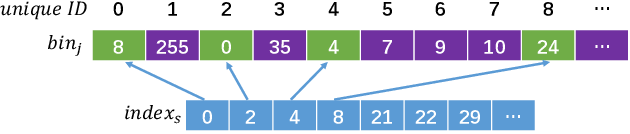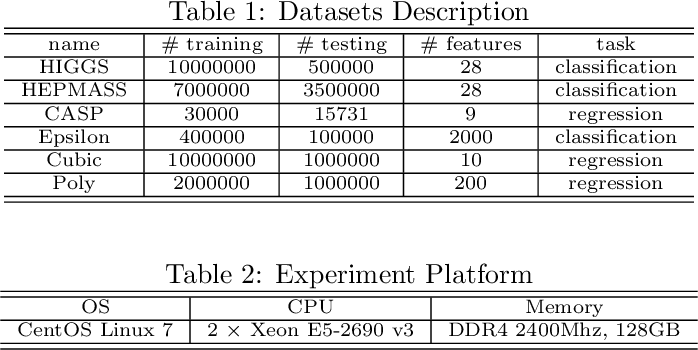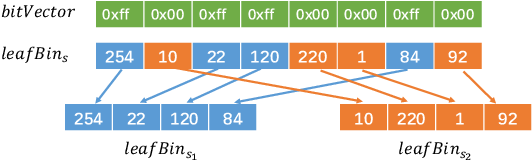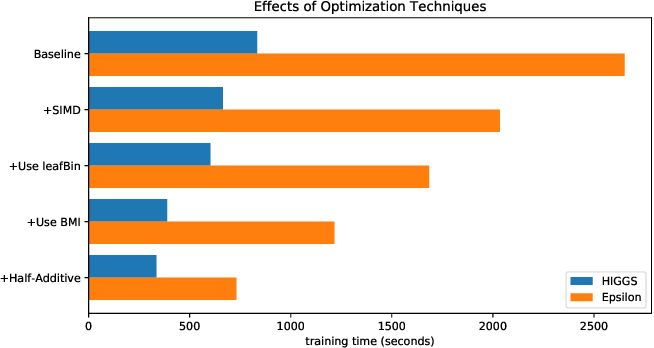Gradient boosting using decision trees as base learners, so called Gradient Boosted Decision Trees (GBDT), is a very successful ensemble learning algorithm widely used across a variety of applications. Recently, various GDBT construction algorithms and implementation have been designed and heavily optimized in some very popular open sourced toolkits such as XGBoost and LightGBM. In this paper, we show that both the accuracy and efficiency of GBDT can be further enhanced by using more complex base learners. Specifically, we extend gradient boosting to use piecewise linear regression trees (PL Trees), instead of piecewise constant regression trees. We show PL Trees can accelerate convergence of GBDT. Moreover, our new algorithm fits better to modern computer architectures with powerful Single Instruction Multiple Data (SIMD) parallelism. We propose optimization techniques to speedup our algorithm. The experimental results show that GBDT with PL Trees can provide very competitive testing accuracy with comparable or less training time. Our algorithm also produces much concise tree ensembles, thus can often reduce testing time costs.

Click to Read Paper and Get Code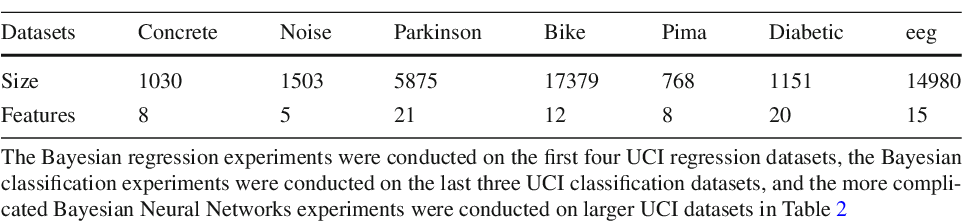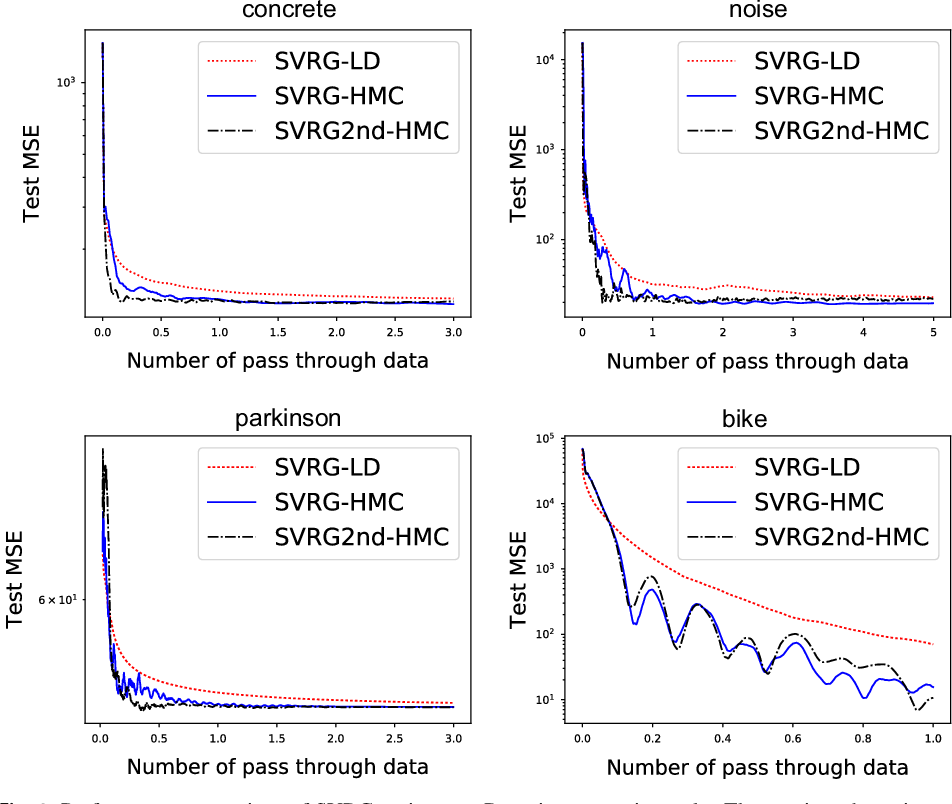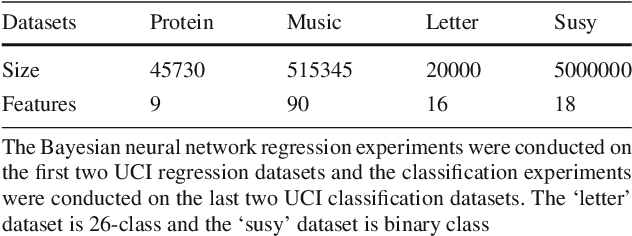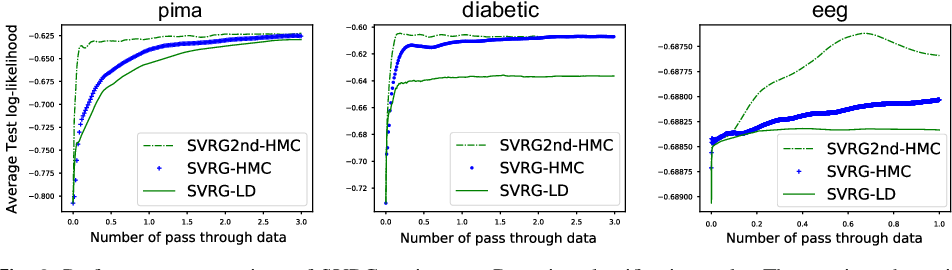Gradient-based Monte Carlo sampling algorithms, like Langevin dynamics and Hamiltonian Monte Carlo, are important methods for Bayesian inference. In large-scale settings, full-gradients are not affordable and thus stochastic gradients evaluated on mini-batches are used as a replacement. In order to reduce the high variance of noisy stochastic gradients, [Dubey et al., 2016] applied the standard variance reduction technique on stochastic gradient Langevin dynamics and obtained both theoretical and experimental improvements. In this paper, we apply the variance reduction tricks on Hamiltonian Monte Carlo and achieve better theoretical convergence results compared with the variance-reduced Langevin dynamics. Moreover, we apply the symmetric splitting scheme in our variance-reduced Hamiltonian Monte Carlo algorithms to further improve the theoretical results. The experimental results are also consistent with the theoretical results. As our experiment shows, variance-reduced Hamiltonian Monte Carlo demonstrates better performance than variance-reduced Langevin dynamics in Bayesian regression and classification tasks on real-world datasets.

* 20 pages
Click to Read Paper and Get Code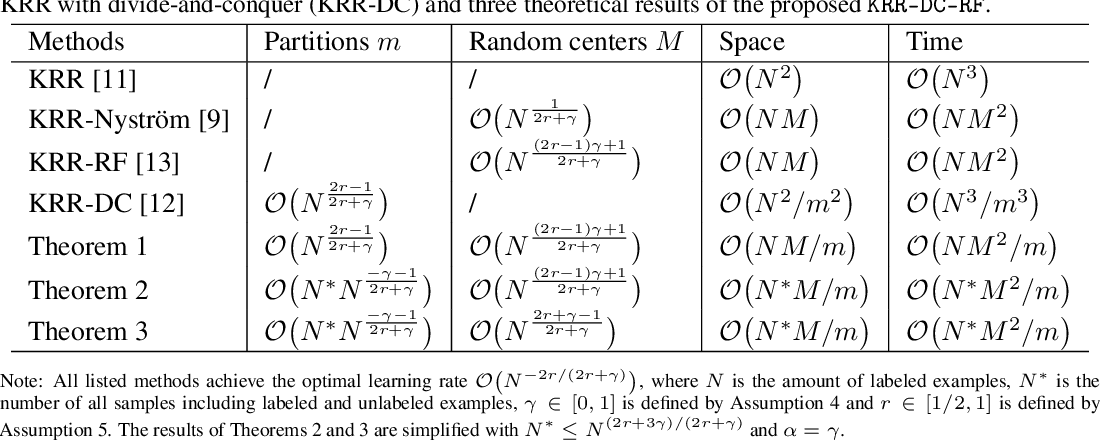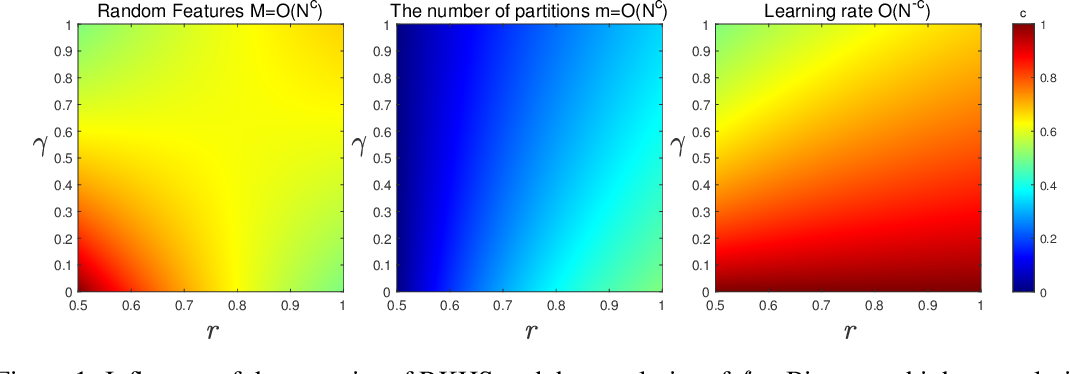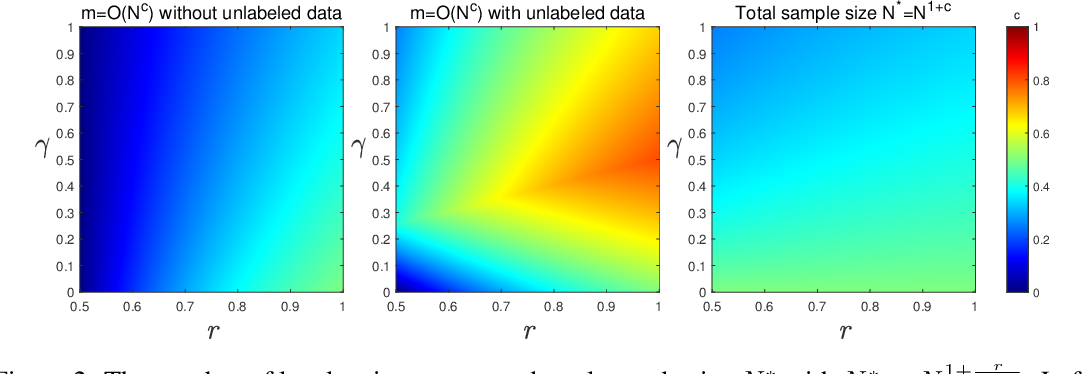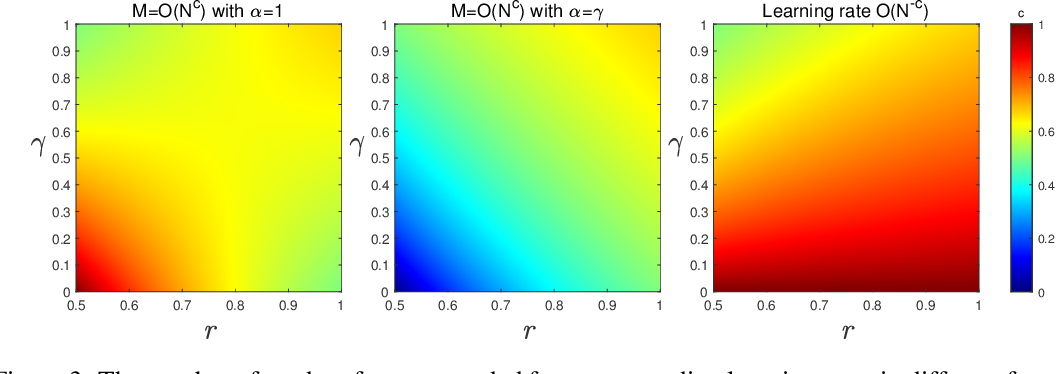Distributed learning and random projections are the most common techniques in large scale nonparametric statistical learning. In this paper, we study the generalization properties of kernel ridge regression using both distributed methods and random features. Theoretical analysis shows the combination remarkably reduces computational cost while preserving the optimal generalization accuracy under standard assumptions. In a benign case, $\mathcal{O}(\sqrt{N})$ partitions and $\mathcal{O}(\sqrt{N})$ random features are sufficient to achieve $\mathcal{O}(1/N)$ learning rate, where $N$ is the labeled sample size. Further, we derive more refined results by using additional unlabeled data to enlarge the number of partitions and by generating features in a data-dependent way to reduce the number of random features.

* 21 pages, 6 figures
Click to Read Paper and Get Code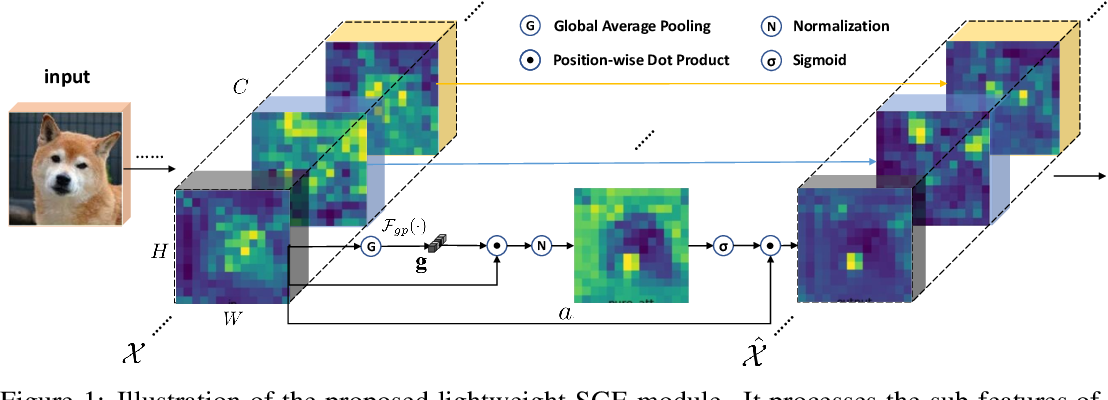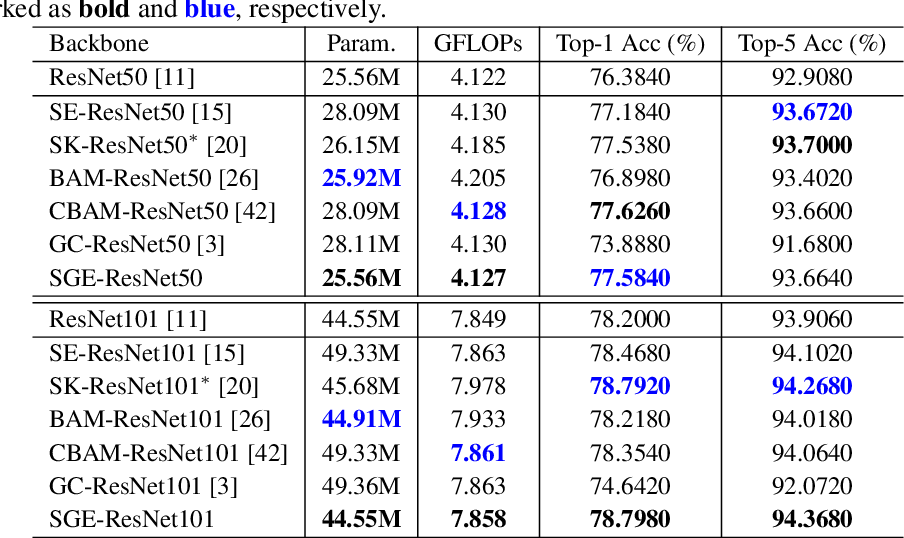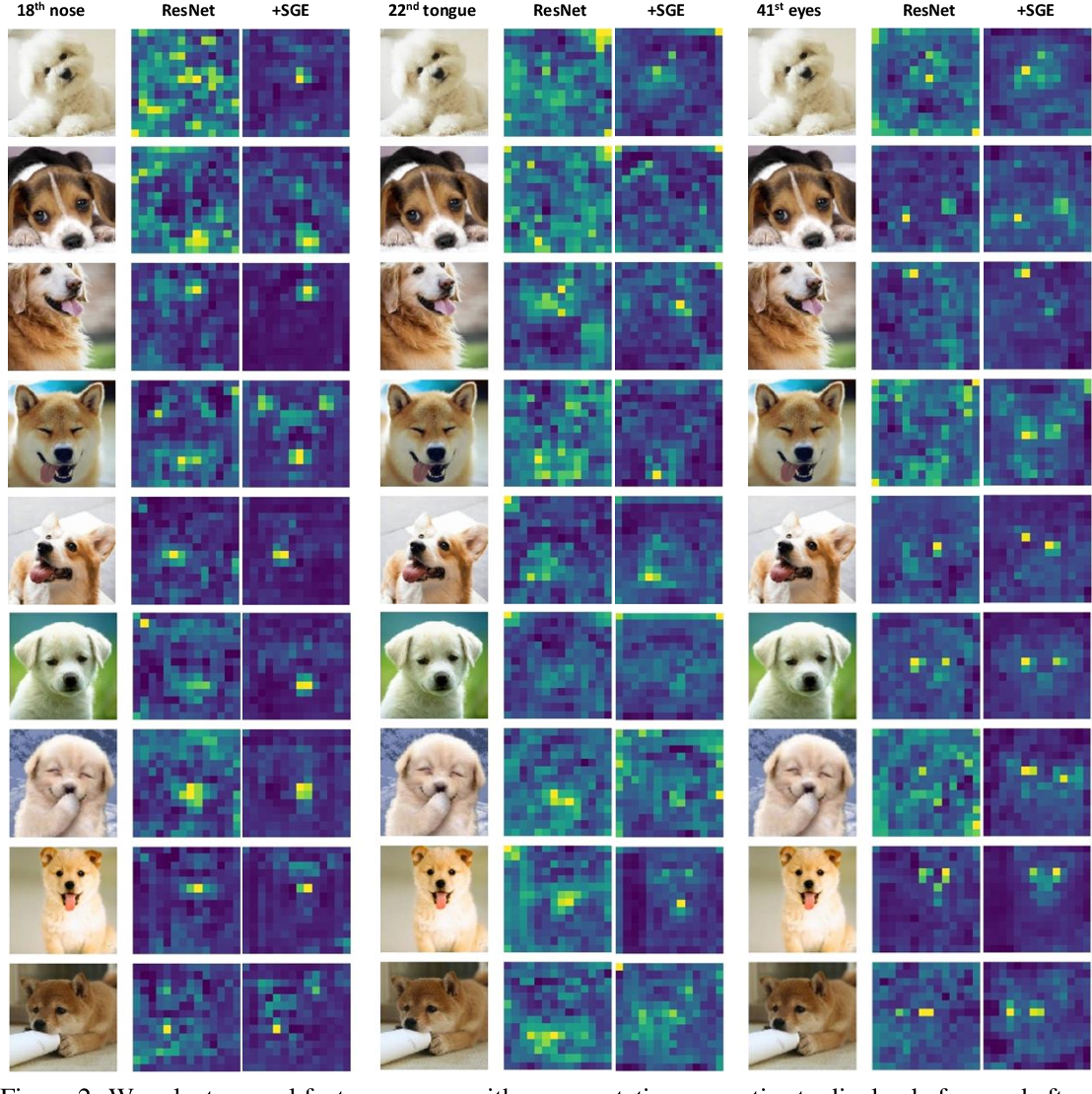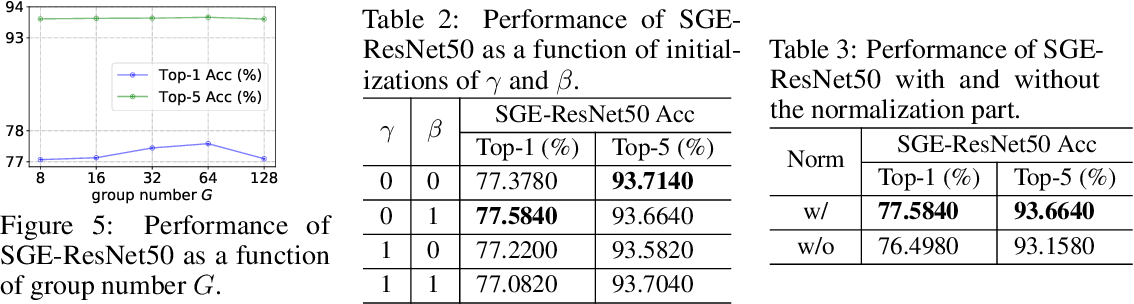The Convolutional Neural Networks (CNNs) generate the feature representation of complex objects by collecting hierarchical and different parts of semantic sub-features. These sub-features can usually be distributed in grouped form in the feature vector of each layer, representing various semantic entities. However, the activation of these sub-features is often spatially affected by similar patterns and noisy backgrounds, resulting in erroneous localization and identification. We propose a Spatial Group-wise Enhance (SGE) module that can adjust the importance of each sub-feature by generating an attention factor for each spatial location in each semantic group, so that every individual group can autonomously enhance its learnt expression and suppress possible noise. The attention factors are only guided by the similarities between the global and local feature descriptors inside each group, thus the design of SGE module is extremely lightweight with \emph{almost no extra parameters and calculations}. Despite being trained with only category supervisions, the SGE component is extremely effective in highlighting multiple active areas with various high-order semantics (such as the dog's eyes, nose, etc.). When integrated with popular CNN backbones, SGE can significantly boost the performance of image recognition tasks. Specifically, based on ResNet50 backbones, SGE achieves 1.2\% Top-1 accuracy improvement on the ImageNet benchmark and 1.0$\sim$2.0\% AP gain on the COCO benchmark across a wide range of detectors (Faster/Mask/Cascade RCNN and RetinaNet). Codes and pretrained models are available at https://github.com/implus/PytorchInsight.

* Code available at: https://github.com/implus/PytorchInsight
Click to Read Paper and Get Code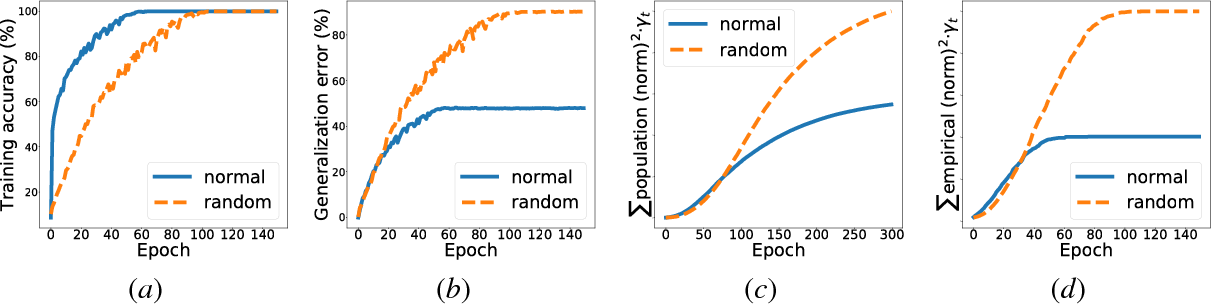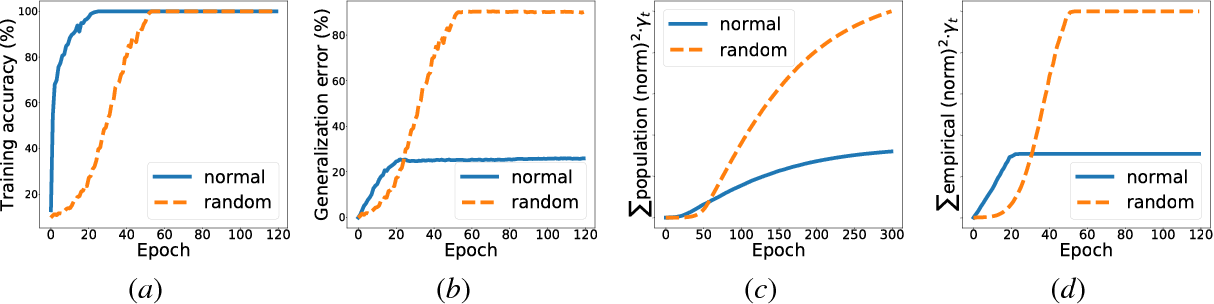Generalization error (also known as the out-of-sample error) measures how well the hypothesis obtained from the training data can generalize to previously unseen data. Obtaining tight generalization error bounds is central to statistical learning theory. In this paper, we study the generalization error bound in learning general non-convex objectives, which has attracted significant attention in recent years. In particular, we study the (algorithm-dependent) generalization bounds of various iterative gradient based methods. (1) We present a very simple and elementary proof of a recent result for stochastic gradient Langevin dynamics (SGLD), due to Mou et al. (2018). Our proof can be easily extended to obtain similar generalization bounds for several other variants of SGLD (e.g., with postprocessing, momentum, mini-batch, acceleration, and more general noises), and improves upon the recent results in Pensia et al. (2018). (2) By incorporating ideas from the PAC-Bayesian theory into the stability framework, we obtain tighter distribution-dependent (or data-dependent) generalization bounds. Our bounds provide an intuitive explanation for the phenomenon reported in Zhang et al. (2017a). (3) We also study the setting where the total loss is the sum of a bounded loss and an additional `l2 regularization term. We obtain new generalization bounds for the continuous Langevin dynamic in this setting by leveraging the tool of Log-Sobolev inequality. Our new bounds are more desirable when the noisy level of the process is not small, and do not grow when T approaches to infinity.

Click to Read Paper and Get Code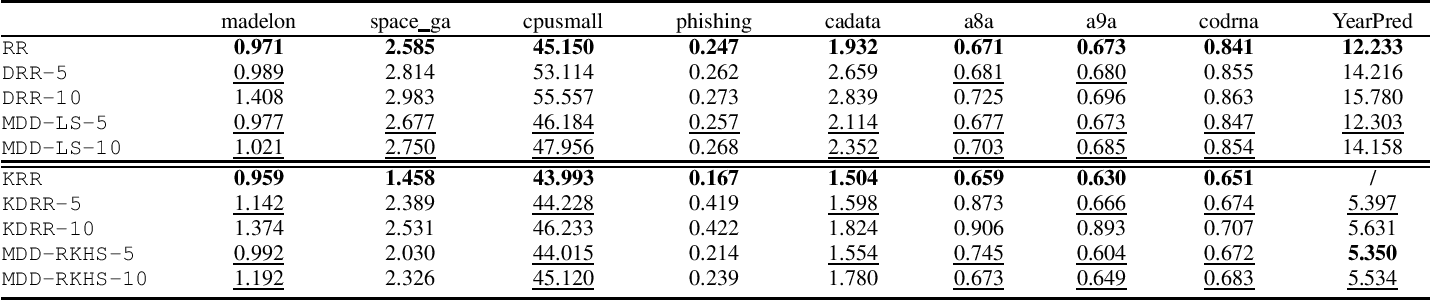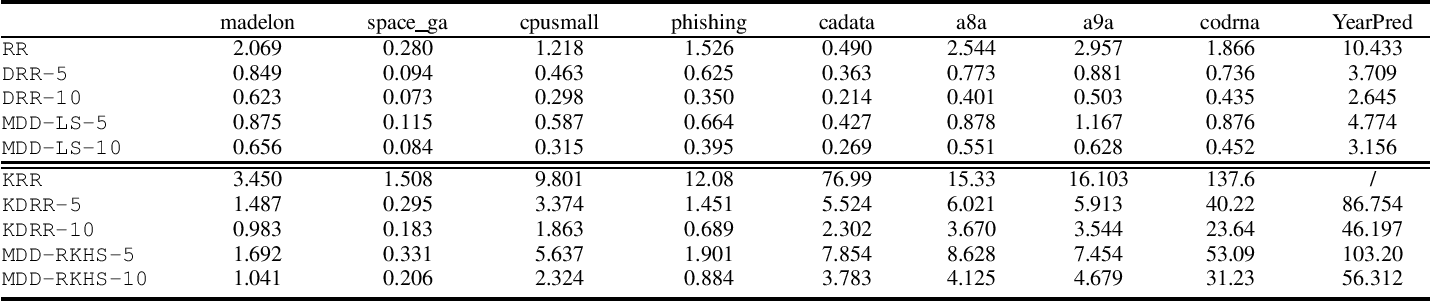We study the risk performance of distributed learning for the regularization empirical risk minimization with fast convergence rate, substantially improving the error analysis of the existing divide-and-conquer based distributed learning. An interesting theoretical finding is that the larger the diversity of each local estimate is, the tighter the risk bound is. This theoretical analysis motivates us to devise an effective maxdiversity distributed learning algorithm (MDD). Experimental results show that MDD can outperform the existing divide-andconquer methods but with a bit more time. Theoretical analysis and empirical results demonstrate that our proposed MDD is sound and effective.

Click to Read Paper and Get Code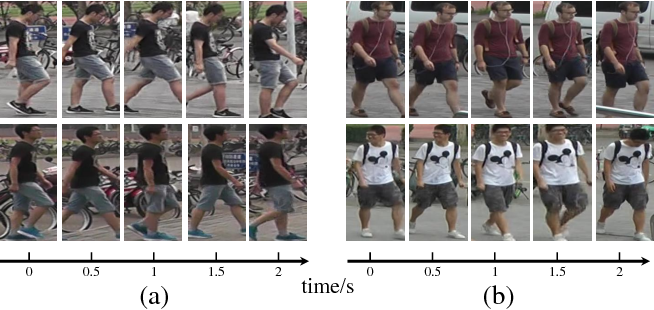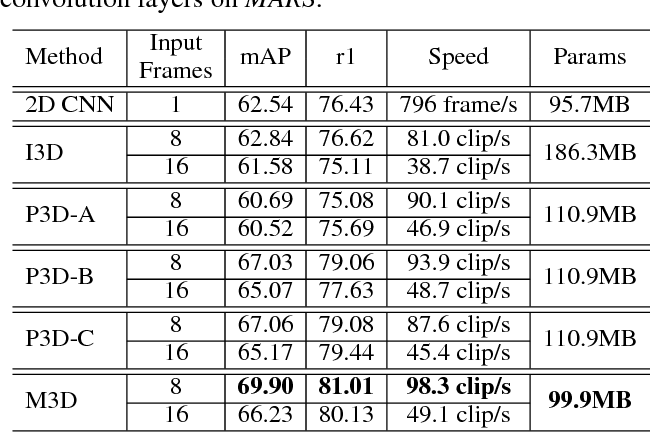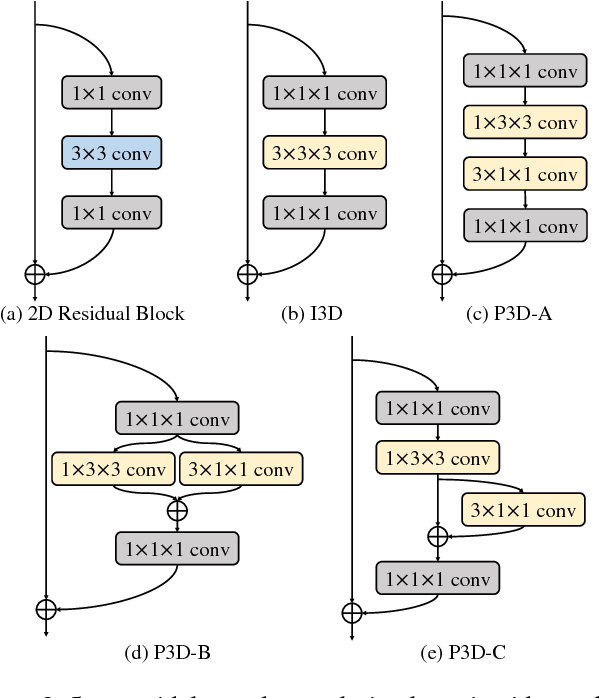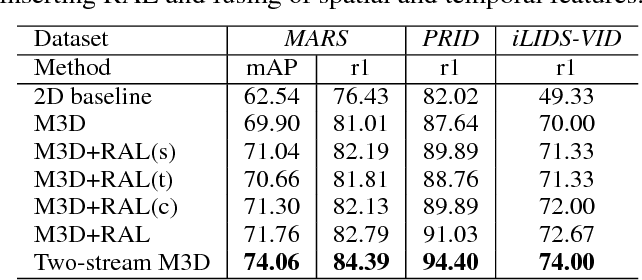This paper proposes a two-stream convolution network to extract spatial and temporal cues for video based person Re-Identification (ReID). A temporal stream in this network is constructed by inserting several Multi-scale 3D (M3D) convolution layers into a 2D CNN network. The resulting M3D convolution network introduces a fraction of parameters into the 2D CNN, but gains the ability of multi-scale temporal feature learning. With this compact architecture, M3D convolution network is also more efficient and easier to optimize than existing 3D convolution networks. The temporal stream further involves Residual Attention Layers (RAL) to refine the temporal features. By jointly learning spatial-temporal attention masks in a residual manner, RAL identifies the discriminative spatial regions and temporal cues. The other stream in our network is implemented with a 2D CNN for spatial feature extraction. The spatial and temporal features from two streams are finally fused for the video based person ReID. Evaluations on three widely used benchmarks datasets, i.e., MARS, PRID2011, and iLIDS-VID demonstrate the substantial advantages of our method over existing 3D convolution networks and state-of-art methods.

* AAAI, 2019
Click to Read Paper and Get Code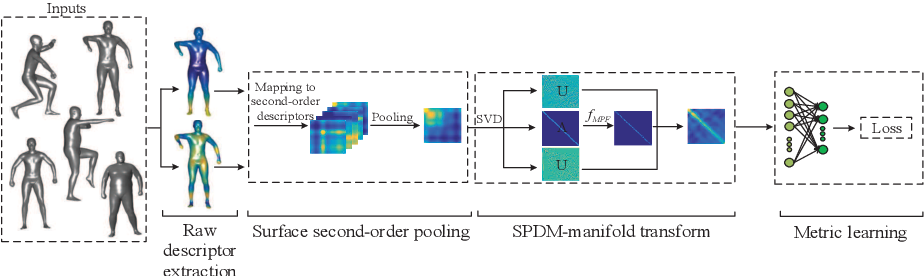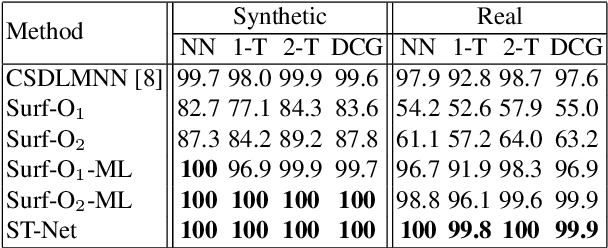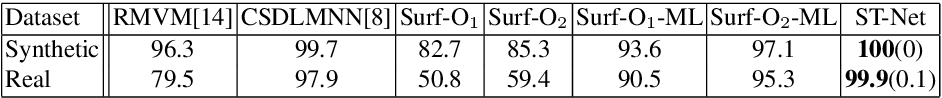Designing a network on 3D surface for non-rigid shape analysis is a challenging task. In this work, we propose a novel spectral transform network on 3D surface to learn shape descriptors. The proposed network architecture consists of four stages: raw descriptor extraction, surface second-order pooling, mixture of power function-based spectral transform, and metric learning. The proposed network is simple and shallow. Quantitative experiments on challenging benchmarks show its effectiveness for non-rigid shape retrieval and classification, e.g., it achieved the highest accuracies on SHREC14, 15 datasets as well as the Range subset of SHREC17 dataset.

* 16 pages, 3 figures
Click to Read Paper and Get Code